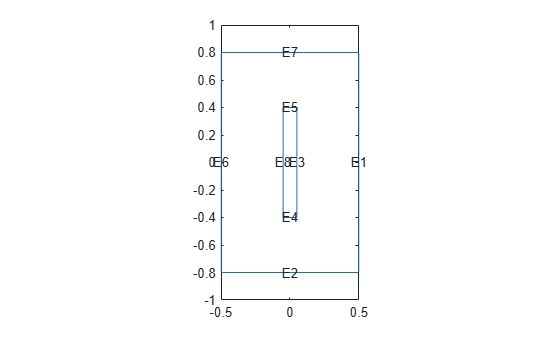# linearizeOutput

Specify outputs of linearized model

Since R2021b

## Syntax

``linearizeOutput(model,RegionType,RegionID)``
``linearizeOutput(model,RegionType,RegionID,"Component",xyz)``
``output = linearizeOutput(___)``

## Description

example

````linearizeOutput(model,RegionType,RegionID)` adds all degrees of freedom (DoFs) associated with the region defined by `RegionType` and `RegionID` to the output vector of the linearized model. For 3-D structural models, `linearizeOutput` adds all x-coordinates first, then all y-coordinates, then all z-coordinates. In the linearized model `sys`, use `sys.OutputGroup` to locate the sections associated with each coordinate.Use `linearizeInput` and `linearizeOutput` together with the `linearize` function to extract sparse linear models from structural and thermal models.```

example

````linearizeOutput(model,RegionType,RegionID,"Component",xyz)` specifies which of the coordinates to include.```
````output = linearizeOutput(___)` returns a structure array `output` with the linearization output description. Use this syntax with any of the previous arguments.```

## Examples

collapse all

Specify the regions of a 2-D thermal model for which `linearize` extracts sparse linear models used in Control System Toolbox™.

Create a transient thermal model.

`thermalmodel = createpde("thermal","transient");`

Add the block geometry to the thermal model by using the `geometryFromEdges` function. The geometry description file for this problem is called `crackg.m`.

`geometryFromEdges(thermalmodel,@crackg);`

Plot the geometry, displaying edge labels.

```pdegplot(thermalmodel,"EdgeLabels","on") ylim([-1,1]) axis equal```Generate a mesh.

`generateMesh(thermalmodel);`

Specify the thermal conductivity, mass density, and specific heat of the material.

```thermalProperties(thermalmodel,"ThermalConductivity",1, ... "MassDensity",1, ... "SpecificHeat",1); ```

Specify the temperature on the left edge as `100`, and constant heat flow to the exterior through the right edge as `-10`. Add a unique label to each boundary condition.

```thermalBC(thermalmodel,"Edge",6,"Temperature",100,"Label","TempBC"); thermalBC(thermalmodel,"Edge",1,"HeatFlux",-10,"Label","FluxBC");```

Specify that the entire geometry generates heat and add a unique label to this assignment.

`internalHeatSource(thermalmodel,25,"Label","HeatSource");`

Set an initial value of `0` for the temperature.

`thermalIC(thermalmodel,0);`

Call the `linearizeInput` function with the previously defined labels for the boundary conditions and the internal heat source to set the inputs for the `linearize` function. Add one label per function call.

```linearizeInput(thermalmodel,"HeatSource"); linearizeInput(thermalmodel,"TempBC"); linearizeInput(thermalmodel,"FluxBC");```

Call the `linearizeOutput` function to specify the regions for which you want `linearize` to extract sparse linear models. Specify one region per function call.

`linearizeOutput(thermalmodel,"Edge",2)`
```ans = struct with fields: RegionType: 'Edge' RegionID: 2 ```

Specify which of the x-, y-, and z- coordinates to include in a linearized model.

Create a structural transient analysis model.

`structuralmodel = createpde("structural","transient-solid");`

Import and plot the tuning fork geometry.

```importGeometry(structuralmodel,"TuningFork.stl"); pdegplot(structuralmodel)```Generate a mesh.

`generateMesh(structuralmodel,"Hmax",0.005);`

Specify Young's modulus, Poisson's ratio, and the mass density to model linear elastic material behavior. Specify all physical properties in consistent units.

```structuralProperties(structuralmodel,"YoungsModulus",210E9, ... "PoissonsRatio",0.3, ... "MassDensity",8000);```

Identify faces for applying boundary constraints and loads by plotting the geometry with the face labels.

```figure("units","normalized","outerposition",[0 0 1 1]) pdegplot(structuralmodel,"FaceLabels","on") view(-50,15) title("Geometry with Face Labels")```Impose sufficient boundary constraints to prevent rigid body motion under applied loading. Typically, you hold a tuning fork by hand or mount it on a table. As a simplified approximation of this boundary condition, fix a region near the intersection of the tines and the handle (faces 21 and 22).

`structuralBC(structuralmodel,"Face",[21,22],"Constraint","fixed");`

Specify the pressure loading on a tine as a short rectangular pressure pulse.

```structuralBoundaryLoad(structuralmodel,"Face",11,... "Pressure",5E6, ... "EndTime",1e-3, ... "Label","Pressure");```

Specify acceleration due to gravity as a body load.

```structuralBodyLoad(structuralmodel, ... "GravitationalAcceleration",[0 0 -1], ... "Label","Gravity");```

Create inputs for gravity and the pressure pulse on tuning fork.

```linearizeInput(structuralmodel,"Gravity"); linearizeInput(structuralmodel,"Pressure");```

Measure the y-displacement of face 12 and x-displacement of face 6.

`linearizeOutput(structuralmodel,"Face",12,"Component","y")`
```ans = struct with fields: RegionType: 'Face' RegionID: 12 Component: 'y' ```
`linearizeOutput(structuralmodel,"Face",6,"Component","x")`
```ans = struct with fields: RegionType: 'Face' RegionID: 6 Component: 'x' ```

## Input Arguments

collapse all

Structural or linear thermal model, specified as a `StructuralModel` object or a `ThermalModel` object. The `linearize` function does not support nonlinear thermal analysis.

Geometric region type, specified as `"Cell"` (for a 3-D model only), `"Face"`, `"Edge"`, or `"Vertex"`.

Data Types: `char`

Geometric region ID, specified as a positive integer. Find the region IDs by using `pdegplot` with the `"CellLabels"`, `"FaceLabels"`, `"EdgeLabels"`, or `"VertexLabels"` value set to `"on"`.

Data Types: `double`

Coordinates to include, specified as a character vector or a string of x-, y-, and z-coordinates to include.

Example: `linearizeOutput(pdemodel,"Face",10,"Component","xz")` selects the x and z DoFs for face 10

Data Types: `char` | `string`

## Output Arguments

collapse all

Linearization output description, returned as a structure array.

## Version History

Introduced in R2021b